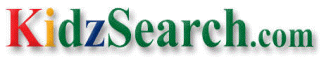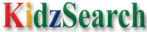2018 - Drawing Lessons For KidsHomeWork : CREATIVE Drawings using Numbers

7:25 AM

HomeWork :

KIDS, you just learned to Draw using Numbers

And now I want you to create a few Drawings using a combination of the Numbers

And yes get it checked from your mommy.

How to draw a ' SEA-HORSE' for kids (Step by Step):

6:51 AM
How to draw ' SEA-HORSE' for kids (Step by Step): Drawings from Number '9' :

LESSON:6

FUN WITH NUMBERS

In this lesson,

we will use numbers to create our drawings

How to draw an ANT for kids (Step by Step):

6:50 AM
How to draw an ANT for kids (Step by Step): Drawings from Number '8' :

LESSON:6

FUN WITH NUMBERS:

In this lesson,

we will use numbers to create our drawings

Number EIGHT  '8' :

How to draw a 'FUNNY CARTOON FACE'for kids (Step by Step):

6:49 AM
How to draw a  'FUNNY CARTOON FACE'for kids (Step by Step): Drawings from Number '7' :

LESSON:6

FUN WITH NUMBERS:

In this lesson,

we will use numbers to create our drawings

Number SEVEN '7' :

How to draw An Elephant face for kids (Step by Step):

6:49 AM
How to draw An Elephant face  for kids (Step by Step): Drawings from Number '6' :

LESSON:6

FUN WITH NUMBERS:

In this lesson,

we will use numbers to create our drawings

Number SIX '6' :

How to draw a FACE of LION for kids (Step by Step):

6:48 AM
How to draw a FACE of LION for kids (Step by Step): Drawings from Number '5' :

LESSON:6

FUN WITH NUMBERS :

In this lesson,
we will use numbers to create our drawings

Number FIVE '5' :

Let's Draw a 'FACE of LION'

How to draw a KITE for kids (Step by Step):

6:47 AM
How to draw a KITE for kids (Step by Step): Drawings from Number '4' :

LESSON:6

FUN WITH NUMBERS

In this lesson,
we will use numbers to create our drawings

How to draw a SQUIRREL for kids (Step by Step):

6:47 AM
How to draw a SQUIRREL for kids (Step by Step): Drawings from Number '3' :

LESSON:6

FUN WITH NUMBERS :

In this lesson,
we will use numbers to create our drawings

How to draw a SWAN for kids (Step by Step):

6:46 AM
How to draw a SWAN for kids (Step by Step): Drawings from Number '2' :

LESSON:6

FUN WITH NUMBERS

In this lesson,
we will use numbers to create our drawings

How to draw a Hand Paper Fan for kids (Step by Step):

6:46 AM
How to draw a Hand Paper Fan for kids (Step by Step): Drawings from Number '1' :

LESSON:6

FUN WITH NUMBERS :

In this lesson,
we will use numbers to create our drawings

How to draw a CLOCK for kids (Step by Step):

6:45 AM
FUN WITH NUMBERS

How to draw a CLOCK for kids (Step by Step): Drawings from Number '0' :

LESSON:6

FUN WITH NUMBERS :

In this lesson,
we will use numbers to create our drawings

Number ZERO '0' :

Let's Draw a Clock

STEP:1

Draw a Circle

STEP:2

Draw an Another Circle inside it

STEP:3

Draw a Curve on top of it

STEP:4

That's it, Drawing is Complete